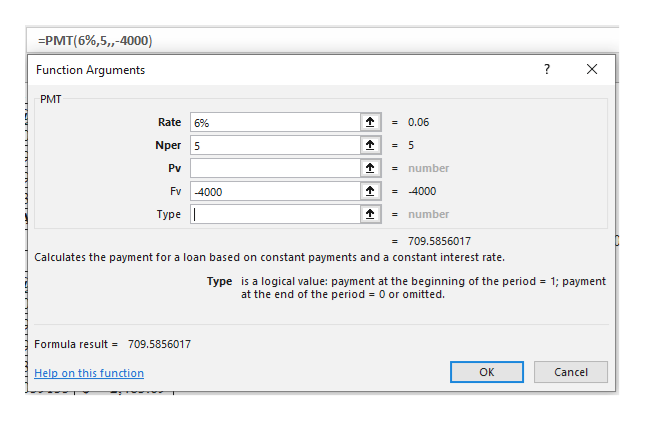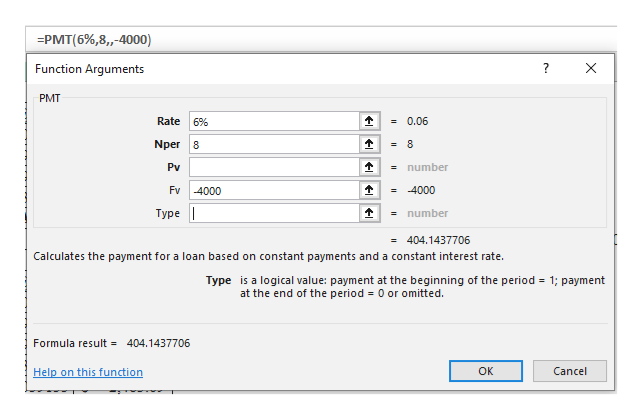How much annual income would need to be generated over a 5 year period, to be equivalent to\$4,000 over an 8 year period? Consider 6% nominal interest (compounded annually) for both cases.

Question
How much annual income would need to be generated over a 5 year period, to be equivalent to
\$4,000 over an 8 year period? Consider 6% nominal interest (compounded annually) for both cases.
Step 1

Calculate the annual income over a period of 5 years as follows:

MS-Excel --> Formulas --> Financials --> PMThelp_outlineImage Transcriptionclose=PMT(6%,5,,-4000) Function Arguments ? X PMT =0.06 Rate 6% = 5 Nper 5 Pv =number Fv =4000 4000 Турe | = number = 709.5856017 Calculates the payment for a loan based on constant payments and a constant interest rate. Type is a logical value: payment at the beginning of the period 1; payment = at the end of the period 0 or omitted. Formula result 709.5856017 Help on this function ок Cancel fullscreen
Step 2

Calculate the annual income over a period of 8 years as follows:

...help_outlineImage Transcriptionclose=PMT(6%,8,-4000) Function Arguments X РMT Rate 6% =0.06 = 8 Nper 8 Pv =number Fv 4000 = 4000 Турe | number = 404.1437706 Calculates the payment for a loan based on constant payments and a constant interest rate. Type is a logical value: payment at the beginning of the period at the end of the period 0 or omitted. 1; payment = 404.1437706 Formula result Cancel OK Help on this function fullscreen

Want to see the full answer?

See Solution

Want to see this answer and more?

Our solutions are written by experts, many with advanced degrees, and available 24/7

See Solution
Tagged in